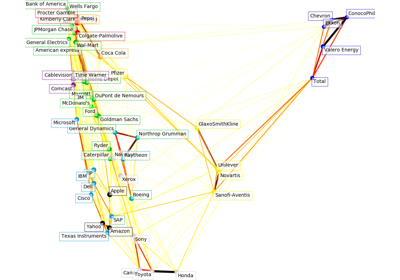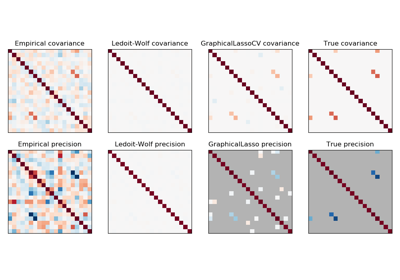# sklearn.covariance.GraphicalLassoCV¶

class sklearn.covariance.GraphicalLassoCV(alphas=4, n_refinements=4, cv='warn', tol=0.0001, enet_tol=0.0001, max_iter=100, mode='cd', n_jobs=None, verbose=False, assume_centered=False)[source]

Sparse inverse covariance w/ cross-validated choice of the l1 penalty.

See glossary entry for cross-validation estimator.

Read more in the User Guide.

Parameters: alphas : integer, or list positive float, optional If an integer is given, it fixes the number of points on the grids of alpha to be used. If a list is given, it gives the grid to be used. See the notes in the class docstring for more details. n_refinements : strictly positive integer The number of times the grid is refined. Not used if explicit values of alphas are passed. cv : int, cross-validation generator or an iterable, optional Determines the cross-validation splitting strategy. Possible inputs for cv are: None, to use the default 3-fold cross-validation, integer, to specify the number of folds. CV splitter, An iterable yielding (train, test) splits as arrays of indices. For integer/None inputs KFold is used. Refer User Guide for the various cross-validation strategies that can be used here. Changed in version 0.20: cv default value if None will change from 3-fold to 5-fold in v0.22. tol : positive float, optional The tolerance to declare convergence: if the dual gap goes below this value, iterations are stopped. enet_tol : positive float, optional The tolerance for the elastic net solver used to calculate the descent direction. This parameter controls the accuracy of the search direction for a given column update, not of the overall parameter estimate. Only used for mode=’cd’. max_iter : integer, optional Maximum number of iterations. mode : {‘cd’, ‘lars’} The Lasso solver to use: coordinate descent or LARS. Use LARS for very sparse underlying graphs, where number of features is greater than number of samples. Elsewhere prefer cd which is more numerically stable. n_jobs : int or None, optional (default=None) number of jobs to run in parallel. None means 1 unless in a joblib.parallel_backend context. -1 means using all processors. See Glossary for more details. verbose : boolean, optional If verbose is True, the objective function and duality gap are printed at each iteration. assume_centered : boolean If True, data are not centered before computation. Useful when working with data whose mean is almost, but not exactly zero. If False, data are centered before computation. covariance_ : numpy.ndarray, shape (n_features, n_features) Estimated covariance matrix. precision_ : numpy.ndarray, shape (n_features, n_features) Estimated precision matrix (inverse covariance). alpha_ : float Penalization parameter selected. cv_alphas_ : list of float All penalization parameters explored. grid_scores_ : 2D numpy.ndarray (n_alphas, n_folds) Log-likelihood score on left-out data across folds. n_iter_ : int Number of iterations run for the optimal alpha.

Notes

The search for the optimal penalization parameter (alpha) is done on an iteratively refined grid: first the cross-validated scores on a grid are computed, then a new refined grid is centered around the maximum, and so on.

One of the challenges which is faced here is that the solvers can fail to converge to a well-conditioned estimate. The corresponding values of alpha then come out as missing values, but the optimum may be close to these missing values.

Methods

 error_norm(comp_cov[, norm, scaling, squared]) Computes the Mean Squared Error between two covariance estimators. fit(X[, y]) Fits the GraphicalLasso covariance model to X. get_params([deep]) Get parameters for this estimator. get_precision() Getter for the precision matrix. mahalanobis(X) Computes the squared Mahalanobis distances of given observations. score(X_test[, y]) Computes the log-likelihood of a Gaussian data set with self.covariance_ as an estimator of its covariance matrix. set_params(**params) Set the parameters of this estimator.
__init__(alphas=4, n_refinements=4, cv='warn', tol=0.0001, enet_tol=0.0001, max_iter=100, mode='cd', n_jobs=None, verbose=False, assume_centered=False)[source]
error_norm(comp_cov, norm='frobenius', scaling=True, squared=True)[source]

Computes the Mean Squared Error between two covariance estimators. (In the sense of the Frobenius norm).

Parameters: comp_cov : array-like, shape = [n_features, n_features] The covariance to compare with. norm : str The type of norm used to compute the error. Available error types: - ‘frobenius’ (default): sqrt(tr(A^t.A)) - ‘spectral’: sqrt(max(eigenvalues(A^t.A)) where A is the error (comp_cov - self.covariance_). scaling : bool If True (default), the squared error norm is divided by n_features. If False, the squared error norm is not rescaled. squared : bool Whether to compute the squared error norm or the error norm. If True (default), the squared error norm is returned. If False, the error norm is returned. The Mean Squared Error (in the sense of the Frobenius norm) between self and comp_cov covariance estimators.
fit(X, y=None)[source]

Fits the GraphicalLasso covariance model to X.

Parameters: X : ndarray, shape (n_samples, n_features) Data from which to compute the covariance estimate y : (ignored)
get_params(deep=True)[source]

Get parameters for this estimator.

Parameters: deep : boolean, optional If True, will return the parameters for this estimator and contained subobjects that are estimators. params : mapping of string to any Parameter names mapped to their values.
get_precision()[source]

Getter for the precision matrix.

Returns: precision_ : array-like The precision matrix associated to the current covariance object.
grid_scores

DEPRECATED: Attribute grid_scores was deprecated in version 0.19 and will be removed in 0.21. Use grid_scores_ instead

mahalanobis(X)[source]

Computes the squared Mahalanobis distances of given observations.

Parameters: X : array-like, shape = [n_samples, n_features] The observations, the Mahalanobis distances of the which we compute. Observations are assumed to be drawn from the same distribution than the data used in fit. dist : array, shape = [n_samples,] Squared Mahalanobis distances of the observations.
score(X_test, y=None)[source]

Computes the log-likelihood of a Gaussian data set with self.covariance_ as an estimator of its covariance matrix.

Parameters: X_test : array-like, shape = [n_samples, n_features] Test data of which we compute the likelihood, where n_samples is the number of samples and n_features is the number of features. X_test is assumed to be drawn from the same distribution than the data used in fit (including centering). y not used, present for API consistence purpose. res : float The likelihood of the data set with self.covariance_ as an estimator of its covariance matrix.
set_params(**params)[source]

Set the parameters of this estimator.

The method works on simple estimators as well as on nested objects (such as pipelines). The latter have parameters of the form <component>__<parameter> so that it’s possible to update each component of a nested object.

Returns: self

## Examples using sklearn.covariance.GraphicalLassoCV¶Visualizing the stock market structureSparse inverse covariance estimation